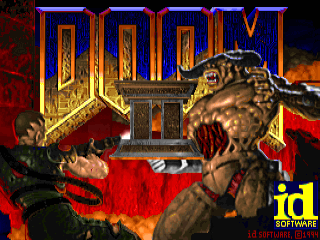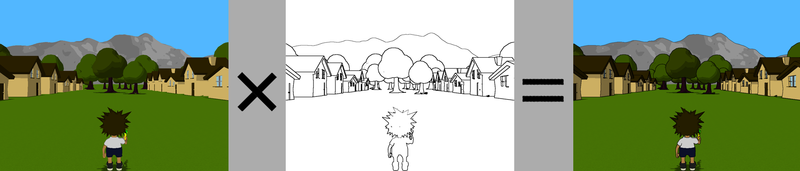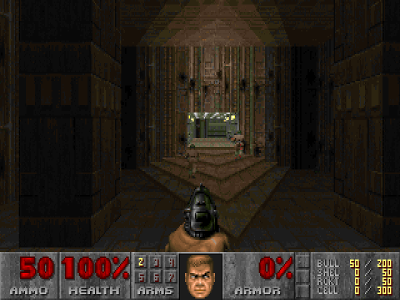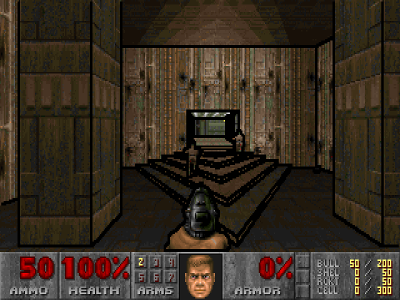Doom (the original version), is not only one of the best game ever made, but it’s also a great example of C code.

Thanks to id Software decision to release the code as open source in 1999, anybody can have a look and modify it.

I had this project of changing the rendering of doom, to add a border effect to the walls, or cel shading. You can check the video at the end of this post to see why I wanted to do this to start with.

This idea of the cel shading algorithm (as described in the Wikipedia page ) is simple: we apply a Sobel filter to both the depth and normal buffers of the rendered frame, that we then superpose to the colors.(Image from wikipedia)

I used the excellent chocolate-doom port of Doom, since this is the closest to the original I could find, and it compiles nicely.

My goal is to create my own buffers for depth and normals, and hook a function to fill them inside the rendering code, then just before the final blit, to call an other function to perform the Sobel filter and applying to the final image.

Here is what it will looks like:Before.After.

The difficulty when looking at doom source is that most of the functions have side effects modifying global variables. I have to admit it is a bit ugly, and I suspect that this was an optimization to avoid useless copies into the stack.

Neither the less, the code is easy to follow, as there is almost no abstractions and indirections. Clearly, John Carmack is an adept of the New Jersey style of programming.

I came up with this simplified function call tree for the rendering algorithm:

``````R_RenderBSPNode
R_Subsector // For each visible subsector
R_FindPlane
R_AddSprites  // Fill the vissprites list
R_AddLine     // For each wall (front to back)
R_ClipSolidWallSegment
R_StoreWallRange(start, stop)
R_CheckPlane
R_RenderSegLoop
R_DrawColumn    // What I am looking for
R_DrawPlanes  // Render ceiling and floor.
``````

What they call ’line’ is really a wall surface. The reason it is a ’line’ is because from a logical point of view, doom walls are just 2d lines extruded vertically (they never have slopes).

As we would expect, the walls are rendered front to back (function `R_AddLine`). To prevent rendering on top of previous walls, the code keeps an array of up to 32 ranges (`struct cliprange`) of already rendered walls. Since the front to back order is guarantied, there is no need for an actual depth buffer.

In my case, what I am looking for is the function `R_DrawColumn`, that ultimately renders a column of pixels from a sprite. It is used for both the walls and the sprites.

Before the function is called, some global variables are set:

``````dc_x:            x position.
dc_yl:           Start y position.
dc_yh:           End y position.
dc_iscale:       Texture scale (i.e: view distance).
rw_normalangle:  Normal of the surface angle.
``````

Those are all I need to maintain the depth and a normal buffers. In `R_DrawColum`, I add a call to my own function `spoom_AddColumn`, that fills some pre-allocated buffers:

``````void spoom_AddColumn(int x, int y1, int y2, int z, int a)
{
double angle = M_PI * a / ANG180;
int y;
for (y = y1; y < y2; y++) {
depthBuffer[y * WIDTH + x] = (double)z / ((uint64_t)1 << 16);
normBuffer[y * WIDTH + x] = cos(angle);
normBuffer[y * WIDTH + x] = sin(angle);
}
}
``````

(Note that at the time when doom was release, such unoptimized code would kill the performances of the game. Fortunately this is of no concern for my needs).

Now that my buffers are filled, I just need to process them with a Sobel filter at each frame, and use the result to add the borders on top of the color buffer. I do it in a single function:

``````// Screen point to the color buffer.
void spoom_Apply(byte *screen)
{
int i, k;
gaussianBlur(depthBuffer, tmpBuffers);
sobel(depthBuffer, tmpBuffers);
gaussianBlur(normBuffer, tmpBuffers);
sobel(normBuffer, tmpBuffers);
gaussianBlur(normBuffer, tmpBuffers);
sobel(normBuffer, tmpBuffers);
for (i = 0; i < WIDTH * HEIGHT; i++) {
if (    depthBuffer[i] > 1.0 ||
normBuffer[i] > 0.5 ||
normBuffer[i] > 0.5) {
screen[i] = 0;
}
}
}
``````

The Sobel and Gaussian filters are implemented with a convolution product:

``````static void convolve(const double *m, const double *kern, double *dst)
{
int i, j, i2, j2;
double v;
for (i = 1; i < HEIGHT - 1; i++)
for (j = 1; j < WIDTH - 1; j++) {
v = 0;
for (i2 = -1; i2 <= 1; i2++)
for (j2 = -1; j2 <= 1; j2++) {
v += m[(i + i2) * WIDTH + j + j2] * kern[(i2 + 1) * 3 + j2 + 1];
}
dst[i * WIDTH + j] = v;
}
}

static void gaussianBlur(double *m, double *tmp)
{
const double kern[] = {
1. / 16, 1. / 8, 1. / 16,
1. /  8, 1. / 4, 1. / 8,
1. / 16, 1. / 8, 1. / 16,
};
convolve(m, kern, tmp);
convolve(tmp, kern, m);
}

static void sobel(double *m, double *tmp)
{
int i;
const double kernx[] = {-1. / 1,  0. / 1,  1. / 1,
-2. / 1,  0. / 1,  2. / 1,
-1. / 1,  0. / 1,  1. / 1};
const double kerny[] = {-1. / 1, -2. / 1, -1. / 1,
0. / 1,  0. / 1,  0. / 1,
1. / 1,  2. / 1,  1. / 1};
convolve(m, kernx, tmp);
convolve(m, kerny, tmp);
for (i = 0; i < WIDTH * HEIGHT; i++)
m[i] = sqrt(tmp[i] * tmp[i] + tmp[i] * tmp[i]);
}
``````

Finally, here is the final result, of me playing doom on a LED POV display. The walls are much easier to see compared to the unmodified game.

By the way, me and a friend are working on this project (link: http://www.spino.tech), feel free to ask us any question about it.